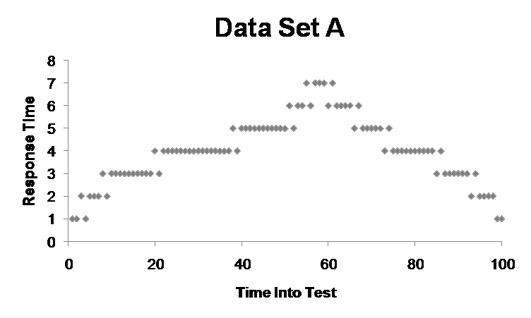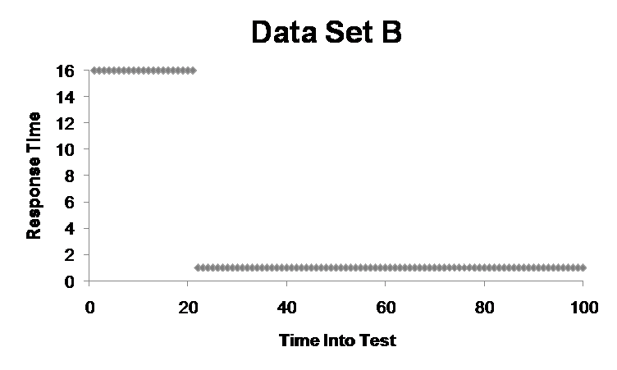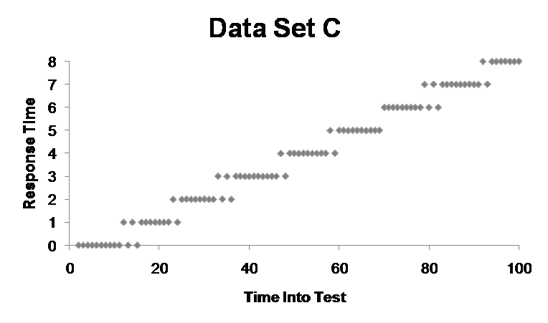{{announcement.body}}
{{announcement.title}}

# 3 Performance Testing Metrics Every Tester Should Know

DZone 's Guide to

# 3 Performance Testing Metrics Every Tester Should Know

### In this post, I want to focus on average, standard deviation and percentiles. We’ll discuss their usefulness when analyzing performance results.

· Performance Zone ·
Free Resource

Comment (3)

Save
{{ articles.views | formatCount}} Views

Making sense of the average, standard deviation and percentiles in performance testing reports.

There are certain performance testing metrics that are essential to understand properly in order to draw the right conclusions from your tests. These metrics require some basic understanding of math and statistics, but nothing too complicated. The issue is that if you don’t understand well what each one means or what they represent, you’ll come to some very wrong conclusions.

In this post, I want to focus on average, standard deviation and percentiles. Without going into a lot of math, we’ll discuss their usefulness when analyzing performance results.

## The Importance of Analyzing Data as a Graph

The first time I thought about this subject was during a course that Scott Barber gave in 2008 (when we were just starting up Abstracta), on his visit to Uruguay. He showed us a table with values like this:He asked us which data set we thought had the best performance, which is not quite as easy to discern as when you display the data in a graph:In Set A, you can tell there was a peak, but then it recovers.In Set B, it seems that it started out with very poor response times and probably 20 seconds into testing, the system collapsed and began to respond to an error page, which then got resolved in a second.Finally, in Set C, it’s clear that as time passed, the system performance continued to degrade.

Barber’s aim with this exercise was to show that it’s much easier to analyze the information when it’s presented in a graph. In addition, in the table, the information is summarized, but in the graphs, you can see all the points. Thus, with more data points, we can gain a clearer picture of what is going on.

## Understanding Key Performance Testing Metrics

Ok, now let’s see what each of the metrics for performance testing means, one by one, and their importance for analysis purposes.

### Average

To calculate the average, simply add up all the values of the samples and then divide that number by the number of samples. Let’s say I do this and my resulting average is 3 seconds. The problem with this is that, at face value, it gives you a false sense that all response times are about three seconds, some a little more and some a little less, but that might not be the case.

Imagine I had three samples, the first two with a response time of one second, the third with a response time of seven:

1 + 1 + 7 = 9

9/3 = 3

This is a very simple example that shows that three very different values could result in an average of three, yet the individual values may not be anywhere close to 3.

My colleague, Fabian Baptista, made a funny comment related to this:

“If I were to put one hand in a bucket of water at -100 degrees Fahrenheit and another hand in a bucket of burning lava, on average, my hand temperature would be fine, but I’d lose both of my hands.”

So, when analyzing average response times, it’s possible to have a result that’s within the acceptable level, but be careful with the conclusions you reach.

That’s why it is not recommended to define service level agreements (SLAs) using averages; instead, have something like “The service must respond in less than 1 second for 99% of cases.” We’ll see more about this later with the percentile metric.

### Standard Deviation

Standard deviation is a measure of dispersion with respect to the average, how much the values vary with respect to their average or how far apart they are. If the value of the standard deviation is small, this indicates that all the values of the samples are close to the average, but if it’s large, then they are far apart and have a greater range.

To understand how to interpret this value, let’s look at a couple of examples.

If all the values are equal, then the standard deviation is 0. If there are very scattered values, for example, consider 9 samples with values from 1 to 9 (1, 2, 3, 4, 5, 6, 7, 8, 9), the standard deviation is ~ 2.6 (you can use this online calculator to calculate it).

Although the value of the average as a metric can be greatly improved by also including the standard deviation, what’s more, useful yet are the percentile values.

### Percentiles

A percentile is a very useful performance testing metric that gives a measure under which a percentage of the sample is found. For example, the 90th percentile (abbreviated as p90) indicates that 90% of the sample is below that value and the rest of the values (that is, the other 10%) are above it.

It’s practical to analyze more than one percentile value (JMeter and Gatling do so in their reports), showing how many samples are p90, p95, and p99. If these are complemented with the minimum, maximum and average, then it’s possible to have a much more complete view of the data and thus understand how the system behaved.

Some percentiles have particular names, such as p100 which is the maximum (100% of the data is below this value), and p50, which is the median (half of the data is below it and half are above).

The percentile is typically used to establish acceptance criteria, indicating that 90% of the sample should be below a certain value. This is done to rule out outliers.

## Careful With Performance Testing Metrics

So, before you go analyzing your next performance test’s results, make sure to remember these key considerations:

• Never consider the average as “the” value to pay attention to, since it can be deceiving, as it often hides important information.
• Consider the standard deviation to know just how useful the average is, the higher the standard deviation, the less meaningful it is.
• Observe the percentile values and define acceptance criteria based on that, keeping in mind that if you select the 90th percentile, you’re basically saying, “I don’t care if 10% of my users experience bad response times”.

What other considerations do you have when analyzing performance testing metrics? Let me know!

Topics:
data, load testing, metrics, performance, performance metrics, quality metrics

Comment (3)

Save
{{ articles.views | formatCount}} Views

Published at DZone with permission of Federico Toledo , DZone MVB. See the original article here.

Opinions expressed by DZone contributors are their own.